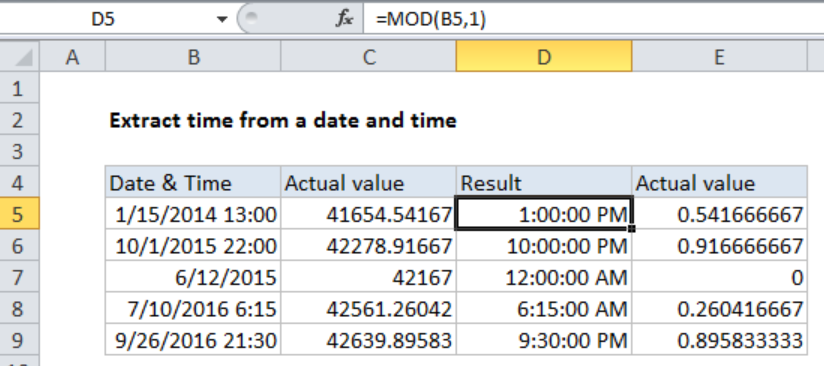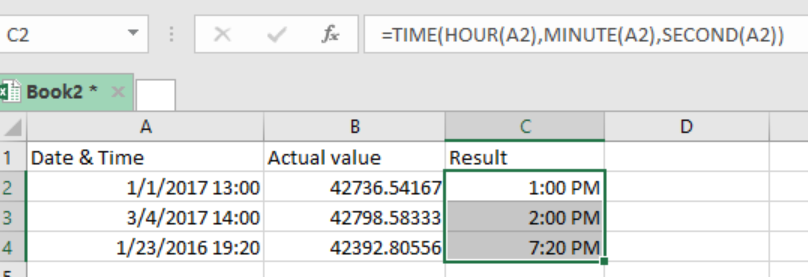Get instant live expert help with Excel or Google Sheets“My Excelchat expert helped me in less than 20 minutes, saving me what would have been 5 hours of work!”

#### Post your problem and you’ll get Expert help in seconds.

Your message must be at least 40 characters
Our professional Expert are available now. Your privacy is guaranteed.

# How to Extract the Time from a Date and Time in Excel

In excel, dates are handled as serial numbers while times as fractional values. There are times when we want to extract time from a date and time. To do this, you will need to use a formula that is based on the MOD function. This article provides an elaborative procedure on how you can use the MOD function to extract time from a date and time in excel.Figure 1: Extracting time from date and time using MOD function

## Syntax of the formula

`=MOD (date, 1)`

Where;

• Date- is the date and time from which you want to extract time.

## Understanding the formula

• MOD function is fundamental when it comes to returning a remainder after division.
• For instance, if the date is in cell B5, then we can have the formula for this as; =MOD(B5,1)
• In this formula, the first argument is always the number, while the second is the divisor.
• MOD will bring the fractional part of the number, which in our case is the date.
• The ability of the MOD function to ignore the integer part and just bring the fractional part makes it the most convenient to extract time from date and time.

The use of the MOD function to extract time from date and time is simply a general format. We can use the formula below to convert the general format to date and time;

`=TIME(HOUR(A2),MINUTE(A2),SECOND(A2))`

This will bring the result as shown in the figure below;Figure 2: Example of how to extract time from date and time using time format

## Instant Connection to an Expert through our Excelchat Service

Most of the time, the problem you will need to solve will be more complex than a simple application of a formula or function. If you want to save hours of research and frustration, try our live Excelchat service! Our Excel Experts are available 24/7 to answer any Excel question you may have. We guarantee a connection within 30 seconds and a customized solution within 20 minutes.

### Did this post not answer your question? Get a solution from connecting with the expert.Another blog reader asked this question today on Excelchat:
Solution examplesI need help with this formula. I need this to include months =INT((M4)/7)&" weeks"&IF(MOD(M4,7)=0,"",", "&INT(MOD(M4,7))&" days") I have the number of Days to Start with
Solved by F. A. in 29 minsneed help with the different sheets and formula IF(A4=B4,0,NETWORKDAYS(A4+1,B4,\$E\$9:\$E\$42) +0.5*(INT((+B4-A4+1)/7)+IF(WEEKDAY(A4+1)+MOD (B4-A4-1,7)>=7,1,0)))
Solved by O. D. in 11 minsi have this formula currently which works but not if I want it for every day - any ideas ?? (NETWORKDAYS.INTL(L1362,M1362,11,BH!A:A)-1)*("17:30"-"8:30")+IF(NETWORKDAYS.INTL(M1362,M1362,11,BH!A:A),MEDIAN(MOD(M1362,1),"8:30","17:30"),"17:30")-MEDIAN(NETWORKDAYS.INTL(L1362,L1362,11,BH!A:A)*MOD(L1362,1),"8:30","17:30")
Solved by A. D. in 28 mins## Subscribe to Excelchat.coAnother blog reader asked this question today on Excelchat: